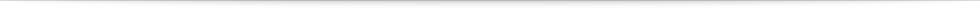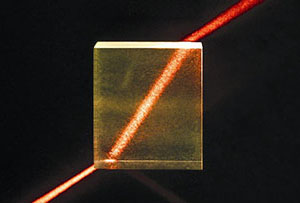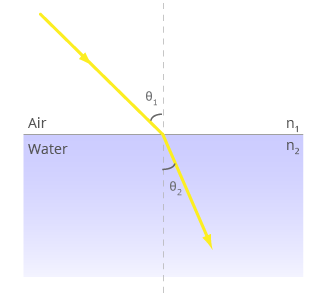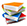Teach
With
Bigshot
 Prev Introduction      Pinhole      Refraction      Lens Basics      Thin Lens Lens Properties      Focusing      Image Appearance      Illustrations Next### RefractionFigure 4: Refraction through a glass slab 

When a ray of light passes from one clear material to another (say, from air to glass or air to water), it bends at the boundary and follows a different direction. This phenomenon, called refraction , can be seen in Figure 4, where a beam of light traveling through air bends as it enters and exits a glass slab.

Refraction is a common occurrence in nature. Without refraction there would not be beautiful rainbows, shimmering mirages or many other spectacular natural phenomena. Another effect of refraction you may have noticed is that swimming pools appear to be shallower than they actually are and their floors look distorted. Our eyes as well as cameras rely on refraction to capture images. So let’s take a closer look at how refraction works.Figure 5: Geometry of refraction

Figure 5 shows a ray of light that is refracted as it passes from air to water. The amount of bending depends on the angle at which the light ray strikes the boundary, as well as the refractive indices  of the two materials (in this case, air and water). The refractive index is simply the bending power of a material. Let us say the refractive indices of air and water are n1 and n2, respectively. Then, according to Snell’s law , a ray coming towards the surface at an angle θ1 will be refracted at an angle θ2 such that:

n1 × sin(θ1) = n2 × sin(θ2).

It is remarkable that this simple equation guides the design of virtually all optical devices, from your reading glasses to camera lenses to powerful telescopes and microscopes. Use the demo in Figure 6 to check out the bending powers of several common materials.

 θ1 θ2 n1 n2 Angle of incidence (θ1=45º) Angle of refraction (θ2=45º) Air (n1 = 1) Water (n1 = 1.33) Glass (n1 = 1.52) Sapphire (n1 = 1.77) Diamond (n1 = 2.4) Air (n2 = 1) Water (n2 = 1.33) Glass (n2 = 1.52) Sapphire (n2 = 1.77) Diamond (n2 = 2.4) Figure 6: Select a material to see how it bends light
 Prev Next###References

  "Light Refraction," [Online image]. Available: http://www.santilli-foundation.org/images/Light-refraction.jpg. [Accessed: Oct 4, 2009].  "Refraction," Wikipedia, The Free Encyclopedia. Oct 2, 2009. [Online]. Available: http://en.wikipedia.org/wiki/Refraction. [Accessed: Oct 4, 2009].  "Refractive index," Wikipedia, The Free Encyclopedia. Oct 2, 2009. [Online]. Available: http://en.wikipedia.org/wiki/Refractive_index. [Accessed: Oct 4, 2009].  "Snell's Law," Wikipedia, The Free Encyclopedia. Oct 2, 2009. [Online]. Available: http://en.wikipedia.org/wiki/Snell's_law. [Accessed: Oct 4, 2009].